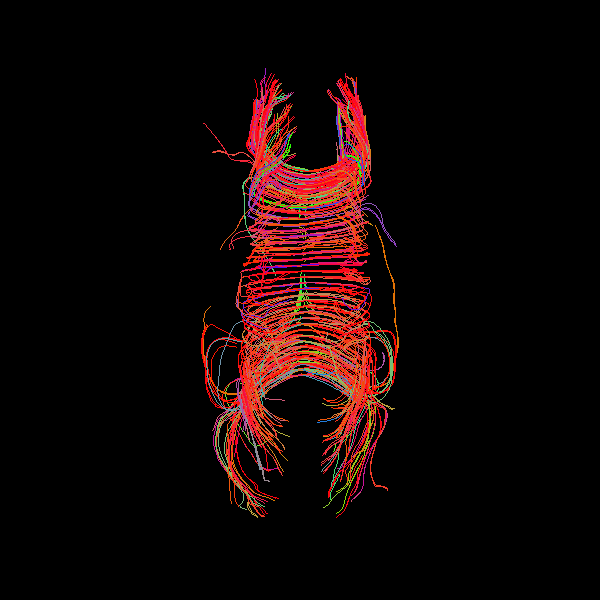# nipy/dipy

Have a question about this project? Sign up for a free GitHub account to open an issue and contact its maintainers and the community.

By clicking “Sign up for GitHub”, you agree to our terms of service and privacy statement. We’ll occasionally send you account related emails.

# tracking fails when npeaks=1 for peaks_from_model with tensor model #1807

Closed
opened this issue Apr 15, 2019 · 1 comment

Contributor

## Description

If you use `peaks_from_model` as the direction getter with a tensor model, setting `npeaks=1`, no streamlines appear when you attempt to visualize. If you set `npeaks=2` (or anything > 1), it visualizes fine. I'm not sure if the problem is in `LocalTracking` or `actor.line`, but this should probably be fixed, this is probably the most intuitive way to run deterministic tractography with a tensor model.

## Way to reproduce

I first noticed this on my own data, and reproduced with the Stanford data:

``````from dipy.data import read_stanford_labels, read_stanford_pve_maps

hardi_img, gtab, labels_img = read_stanford_labels()
data = hardi_img.get_fdata()
labels = labels_img.get_data()
affine = hardi_img.affine
pve_csf, pve_gm, pve_wm = read_stanford_pve_maps()

from dipy.tracking.local import BinaryTissueClassifier
classifier = BinaryTissueClassifier(pve_wm.get_data() > 0)

from dipy.tracking.utils import seeds_from_mask
seed_mask = labels == 2

from dipy.reconst.dti import TensorModel
tensor_model = TensorModel(gtab)

from dipy.data import get_sphere
from dipy.reconst.peaks import peaks_from_model

print('Getting peaks, npeaks=1')
dti_dg_1 = peaks_from_model(model=tensor_model,
data=data,
sphere=get_sphere('repulsion724'),
relative_peak_threshold=.2,
min_separation_angle=25,
mask=(labels == 2) | (labels == 1),
npeaks=1)

print('Tracking')
from dipy.tracking.local import LocalTracking
from dipy.tracking.streamline import Streamlines

streamline_generator_1 = LocalTracking(dti_dg_1,
classifier,
seeds,
hardi_img.affine,
step_size=0.5)
streamlines_1 = Streamlines(streamline_generator_1)

print('Visualizing')
from dipy.viz import window, actor, colormap
ren = window.Renderer()
window.record(ren, out_path='dti_from_peaks_npeaks1.png', size=(600, 600))

print('Getting peaks, npeaks=2')
dti_dg_2 = peaks_from_model(model=tensor_model,
data=data,
sphere=get_sphere('repulsion724'),
relative_peak_threshold=.2,
min_separation_angle=25,
mask=(labels == 2) | (labels == 1),
npeaks=2)

print('Tracking')
from dipy.tracking.local import LocalTracking
from dipy.tracking.streamline import Streamlines

streamline_generator_2 = LocalTracking(dti_dg_2,
classifier,
seeds,
hardi_img.affine,
step_size=0.5)
streamlines_2 = Streamlines(streamline_generator_2)

print('Visualizing')
from dipy.viz import window, actor, colormap
ren = window.Renderer()
window.record(ren, out_path='dti_from_peaks_npeaks2.png', size=(600, 600))
``````Member

### skoudoro commented Jul 12, 2019

 fixed by #1888, closing

###skoudoro closed this Jul 12, 2019

to join this conversation on GitHub. Already have an account? Sign in to comment
Projects
None yet
You can’t perform that action at this time.